# Stress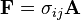${\mathbf F} = \sigma_{ij} {\mathbf A}$
where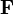${\mathbf F}$ is the force,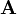${\mathbf A}$ is the area, and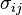$\sigma_{ij}$ is the stress tensor, given by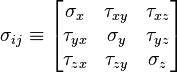$\sigma_{ij} \equiv \left[{\begin{matrix} \sigma _x & \tau _{xy} & \tau _{xz} \\ \tau _{yx} & \sigma _y & \tau _{yz} \\ \tau _{zx} & \tau _{zy} & \sigma _z \\ \end{matrix}}\right]$
where where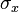$\ \sigma_{x}$,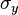$\ \sigma_{y}$, and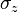$\ \sigma_{z}$ are normal stresses, and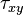$\ \tau_{xy}$,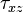$\ \tau_{xz}$,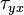$\ \tau_{yx}$,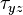$\ \tau_{yz}$,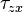$\ \tau_{zx}$, and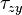$\ \tau_{zy}$ are shear stresess.